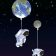# Unique Binary Search Trees

Given n, how many structurally unique BST’s (binary search trees) that store values 1 … n?

Example:

```Input: 3
Output: 5
Explanation:
Given n = 3, there are a total of 5 unique BST's:

1         3     3      2      1
\       /     /      / \      \
3     2     1      1   3      2
/     /       \                 \
2     1         2                 3
```

Solutions:

``class Solution {    public int numTrees(int n) {        int[] count = new int[n+1];        count = 1;        count = 1;        for (int i = 2; i &lt;=n; i++){            for (int j = 1; j &lt;= i; j ++){                count[i] += count[j-1] * count[i-j];            }        }        return count[n];    }}``

## 发布者